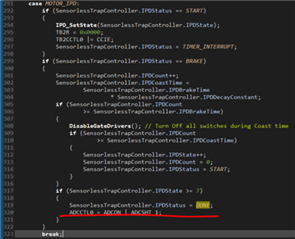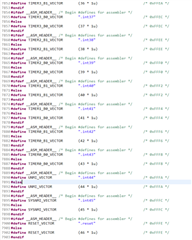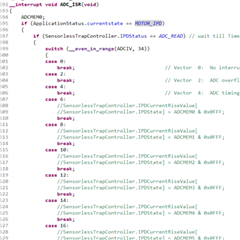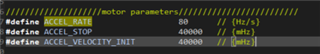If you have a related question, please click the "Ask a related question" button in the top right corner. The newly created question will be automatically linked to this question.

# TIDA-010031: TIDA-010031

Part Number: TIDA-010031
Other Parts Discussed in Thread: MSP430FR2355

Hello.

we have few queries on TIDA board firmware for BLDC motor driver, listed below.

https://dev.ti.com/tirex/explore/node?a=VLyFKFf__1.0.4&node=AHncK.hWJ4vOir8iwD1B1w__coGQ502__LATEST

1- The SensorlessTrapController.IPDStatus is assigned the value ADC_READ in the ISR when the state machine is in MOTOR_IPD state. But then, post this assignment, I don't see this being handled anywhere else in the code. It again looks like the code could reach this point and get stuck here indefinitely. Where is the logic to get out of this state implemented?

2- The below are  vectors being used in the ISR of the motor control software. Not able to find the corresponding counter registers etc. for these vectors anywhere including datasheet.

We are refering to msp430fr2355 datasheet which is used in TIDA board

TIMER0_B0_VECTOR

TIMER2_B0_VECTOR

TIMER2_B1_VECTOR

TIMER3_B0_VECTOR

TIMER3_B1_VECTOR

3-  The logic to convert acceleration to angular distance is implemented this way:

SensorlessTrapController.AccelVelocityInit +=

SensorlessTrapController.AccelRate; //increase velocity by the acceleration rate

SensorlessTrapController.AccelDistance +=

(SensorlessTrapController.AccelVelocityInit

- (SensorlessTrapController.AccelRate >> 1)) >> 3; //calculate distance

What is the physics based derivation for this logic?

Also, in the above code, when SensorlessTrapController.AccelDistance hits the value 20834, it means it's travelled 60 degrees. How is 20834 derived?

4- I have a couple questions on one particular state in the state machine - MOTOR_DIRECTION

1. Where is the logic for MOTOR_DIRECTION implemented? From my perusal of the code, I am not able to find that anywhere?
1. Once the ApplicationStatus.currentstate is set to MOTOR_DIRECTION, how does it get back to a different state? I don't find code anywhere for it to change any state post this assignment. Looks like it might just get stuck there.

• HI

1.You can see the condition of IPDStatus jump in ISR.C2.You can find the corresponding definition in MSP430FR2355.h3.We need to confirm this detail

4.Under normal circumstances, we will not enter the MOTOR_DIRECTION state and have not processed it.

Thanks

Jenson

• Hello Jenson,

Query on answer 1:  The code would only enter this segment when IPDStatus == BRAKE (per the IF statement within which this segment is). But we currently have assigned IPDStatus as ADC_READ. How/where does the code move from IPDStatus of ADC_READ to BRAKE?

Please refer to the following code：Thanks.

Jenson

• Hello Jenson,

Sorry for late.

For this code, the electrical angle of the motor is calculated in an open loop. His physical formula derivation is the calculation formula of speed and distance. We set his acceleration to 80Hz/s. The acceleration is related to the 60° electrical angle. The faster the acceleration, the faster the commutation in the open loop. The same 60° is obtained through conversion, and it determines the commutation speed in open loop together with the acceleration。Thanks

Jenson

• Hello, thank you for your response. But we're looking for some more elaboration. In particular, we're looking to control our motor for a specific application using MSP430FR2355 and we need to modify this code to change the PWM period, acceleration rate etc. depending on our application. We're trying to understand how to calculate these constants (like ACCEL_60_DEGREES for instance). Would you be able to share the physics based formula, or the relation of acceleration rate to 60 degrees angle or the conversion etc?

• Hi Vinay,

The calculation formula is based on ：Convert it to an angle, because 60°=pi/3, the angle value can be obtained according to the calculation

By the way, what company are you, I think the sales team of TI can give you a certain amount of support, so that you can carry out research and development quickly

Thanks

Jenson

• Thank you, Jenson! This helps!

I think someone from my Org is already in touch with TI sales and I think they mentioned that this forum would be the route to get the fastest response.